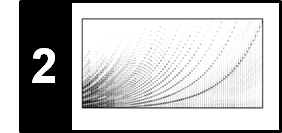# Interference and Beating

Following Section 2.3.3.1 of [Müller, FMP, Springer 2015], we discuss in this notebook the effect of signal interference and the phenomenon of beating.

## Interference¶

In signal processing, interference occurs when a wave is superimposed with another wave of similar frequency. When a crest of one wave meets a crest of the other wave at some point, then the individual magnitudes add up for a certain period of time, which is known as constructive interference. Vice versa, when a crest of one wave meets a trough of the other wave, then the magnitudes cancel out for a certain period of time, which is known as destructive interference.

In :
import numpy as np
from matplotlib import pyplot as plt
import sys
sys.path.append('..')
import libfmp.c1
%matplotlib inline

def plot_interference(x1, x2, t, figsize=(8, 2), xlim=None, ylim=None, title=''):
"""Helper function for plotting two signals and its superposition

Notebook: C2/C2S3_InterferenceBeating.ipynb

Args:
x1: Signal 1
x2: Signal 2
t: Time
figsize: figure size (Default value = (8, 2))
xlim: x limits (Default value = None)
ylim: y limits (Default value = None)
title: figure title (Default value = '')
"""
plt.figure(figsize=figsize)
plt.plot(t, x1, color='gray', linewidth=1.0, linestyle='-', label='x1')
plt.plot(t, x2, color='cyan', linewidth=1.0, linestyle='-', label='x2')
plt.plot(t, x1+x2, color='red', linewidth=2.0, linestyle='-', label='x1+x2')
if xlim is None:
plt.xlim([0, t[-1]])
else:
plt.xlim(xlim)
if ylim is not None:
plt.ylim(ylim)
plt.xlabel('Time (seconds)')
plt.ylabel('Amplitude')
plt.title(title)
plt.legend(loc='upper right')
plt.tight_layout()
plt.show()

dur = 5
x1, t = libfmp.c1.generate_sinusoid(dur=dur, Fs=1000, amp=1, freq=1.05, phase=0.0)
x2, t = libfmp.c1.generate_sinusoid(dur=dur, Fs=1000, amp=1, freq=0.95, phase=0.8)
plot_interference(x1, x2, t, xlim=[0, dur], ylim=[-2.2,2.2], title='Constructive Interference');

dur = 5
x1, t = libfmp.c1.generate_sinusoid(dur=dur, Fs=1000, amp=1, freq=1.05, phase=0.0)
x2, t = libfmp.c1.generate_sinusoid(dur=dur, Fs=1000, amp=1, freq=1.00, phase=0.4)
plot_interference(x1, x2, t, xlim=[0, dur], ylim=[-2.2,2.2], title='Destructive Interference');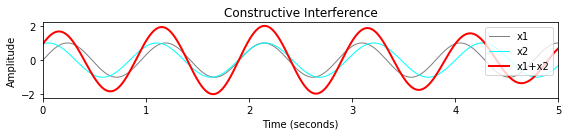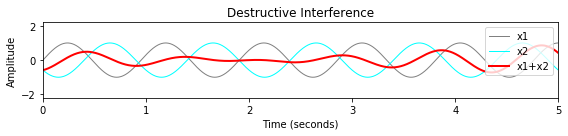## Beating¶

The previous figure shows that two sinusoids of similar frequency may add up (constructive interference) or cancel out (destructive interference). Let $f_1(t)=\sin(2\pi \omega_1 t)$ and $f_2(t)=\sin(2\pi \omega_2 t)$ be two such sinusoids with distinct but nearby frequencies $\omega_1\approx\omega_2$. We now show that the superposition $f_1+f_2$ of these two sinusoids results in a function that looks like a single sine wave with a slowly varying amplitude, a phenomenon also known as beating. Mathematically, this phenomenon results from a trigonometric identity yielding

$$\sin(2\pi \omega_1 t)+\sin(2\pi \omega_2 t)= 2\cos\left(2\pi\frac{\omega_1-\omega_2}{2}t\right)\sin\left(2\pi\frac{\omega_1+\omega_2}{2}t\right).$$

If the difference $\omega_1-\omega_2$ is small, the cosine term has a low frequency compared to the sine term. As a result the signal $f_1+f_2$ can be seen as a sine wave of frequency $(\omega_1+\omega_2)/2$ with a slowly varying amplitude envelope of frequency $|\omega_1-\omega_2|$. Note that this rate is twice the frequency $(\omega_1-\omega_2)/2$ of the cosine term. The following code example illustrates the beating effect.

In :
import IPython.display as ipd
%matplotlib inline

Fs = 4000
dur = 5
x1, t = libfmp.c1.generate_sinusoid(dur=dur, Fs=Fs, amp=0.5, freq=200)
x2, t = libfmp.c1.generate_sinusoid(dur=dur, Fs=Fs, amp=0.5, freq=203)
plot_interference(x1, x2, t, ylim=[-1.1,1.1], xlim=[0, dur],
title=r'Beating with beating frequency $|\omega_1-\omega_2|=3$ ($\omega_1=200, \omega_2=203$)');
plot_interference(x1, x2, t, ylim=[-1.1,1.1], xlim=[1.115, 1.225], title=r'Zoom-in section');

ipd.display(ipd.Audio(x1+x2, rate=Fs))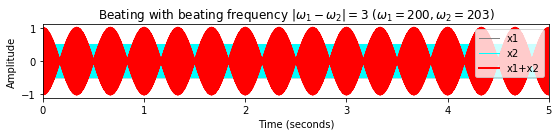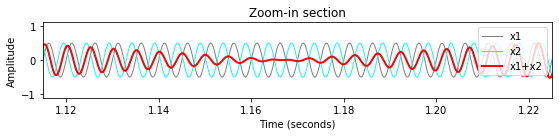## Chirp Experiment¶

To further illustrate the beating effect, we consider a chirp signal (also called sweep signal), in which the frequency increases with time. Let $\omega_0,\omega_1\in\mathbb{R}$ be two frequency parameters (given in Hertz) and $T\in\mathbb{R}$ a duration parameter (given in seconds). A linear chirp of duration $d$ that linearly increases in frequency starting with $\omega_0$ and ending with $\omega_1$ is given by:

$$f(t)=\sin\left(\pi\frac{\omega_1-\omega_0}{T} t^2 + 2\pi\omega_0t\right)$$

for $t\in[0,T]$. Note that the instantaneous frequency of the chirp signal $f$ at time $t$ is given by the derivate of the sinusoid's argument divided by $2\pi$:

$$g(t) = \frac{\omega_1-\omega_0}{T} t + \omega_0.$$

In the following, we consider a linear chirp signal starting with frequency $\omega_0=220.0~\mathrm{Hz}$ (pitch $\mathrm{A3}$) and ending with frequency $\omega_1=311.1~\mathrm{Hz}$ (pitch $\mathrm{E}^\flat 4$) of duration $T=20~\mathrm{sec}$. Furthermore, we consider a sinusoid with frequency $261.5~\mathrm{Hz}$ (pitch $\mathrm{C4}$) of the same duration. When listening to a superposition of these signal, one first perceives the two separate pitches $\mathrm{A3}$ and $\mathrm{C4}$. When the chirp approaches $\mathrm{C4}$, the two pitches begin fusing into a single sound. At the same time, one can notice a beating effect, which first slows down, then disappears (when the chirp reaches $\mathrm{C4}$), and then speeds up again. At the end, one again perceives two pitches, now being $\mathrm{E}^\flat 4$ and $\mathrm{C4}$.

In :
def generate_chirp_linear(dur, freq_start, freq_end, amp=1.0, Fs=22050):
"""Generation chirp with linear frequency increase

Notebook: C2/C2S3_InterferenceBeating.ipynb

Args:
dur (float): Duration (seconds) of the signal
freq_start (float): Start frequency of the chirp
freq_end (float): End frequency of the chirp
amp (float): Amplitude of chirp (Default value = 1.0)
Fs (scalar): Sampling rate (Default value = 22050)

Returns:
x (np.ndarray): Generated chirp signal
t (np.ndarray): Time axis (in seconds)
freq (np.ndarray): Instant frequency (in Hz)
"""
N = int(dur * Fs)
t = np.arange(N) / Fs
a = (freq_end - freq_start) / dur
freq = a * t + freq_start
x = amp * np.sin(np.pi * a * t ** 2 + 2 * np.pi * freq_start * t)
return x, t, freq

f_pitch = lambda p: 440 * 2 ** ((p - 69) / 12)

Fs = 4000
dur = 20
freq_start = f_pitch(57)   # A3
freq_end = f_pitch(63)     # Eflatp4
freq_sin = f_pitch(60)     # C4
x1, t, freq = generate_chirp_linear(dur=dur, freq_start=freq_start, freq_end=freq_end, amp=0.5, Fs=Fs)
x2, t = libfmp.c1.generate_sinusoid(dur=dur, Fs=Fs, amp=0.5, freq=freq_sin)

y = x1 + x2
ipd.display(ipd.Audio(y, rate=Fs))
plot_interference(x1, x2, t, xlim=[0, dur], ylim=[-1.1,1.1],
title=r'Superposition of a linear chirp $x_1$ (A3 to E$^\flat$4) and sinusoid $x_2$ (C4)');
plot_interference(x1, x2, t, xlim=[7, 11], ylim=[-1.1,1.1], title=r'Zoom-in section');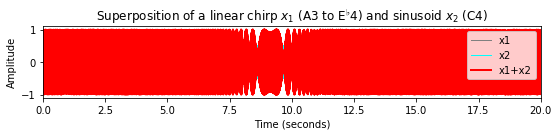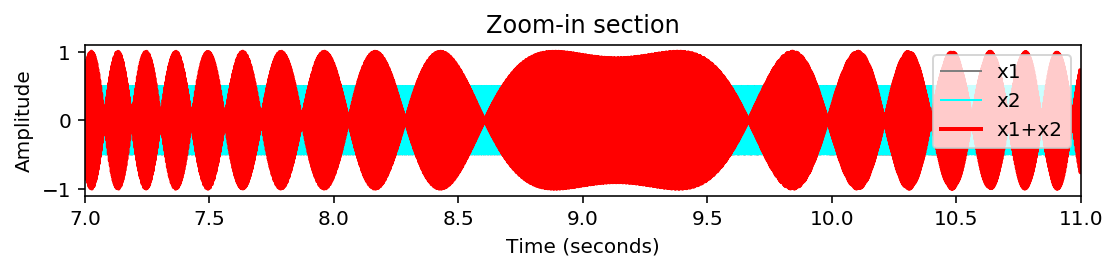Acknowledgment: This notebook was created by Meinard Müller.